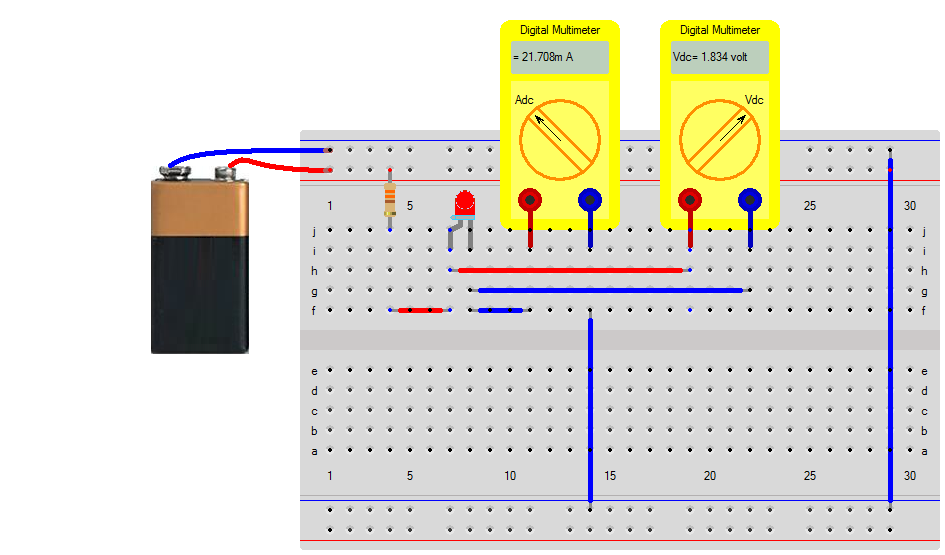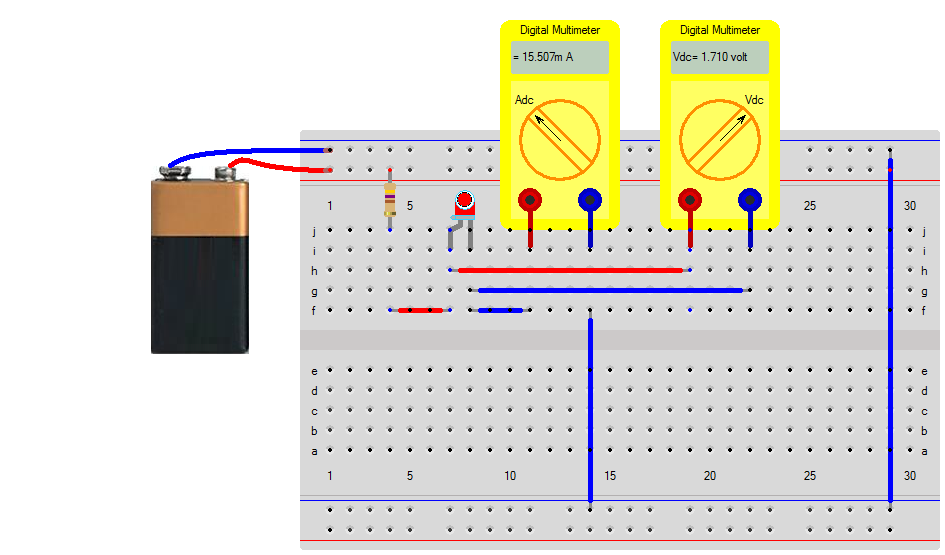## LED current limiting resistor

The key to maximizing LED life is limiting the current that runs through it. This is frequently done with a simple resistor whose value is calculated using Ohm's Law.

When computing the value of a current limiting resistor for a single LED, the basic form of Ohm's Law $$V = I \cdot R$$ becomes $R = \frac{V_{battery} - V_{LED}}{I_{LED}}$

### Resistor of 330Ω

For a 9v battery and a single LED the current can be limited to 20mA as $R=\frac{9v-2v}{20mA} = 350 \Omega$

Choose a 330Ω resistor and assuming only a voltage drop of 1.8 volts across the resistor will give a current as $I=\frac{9v-1.8v}{330\Omega} = 22 mA$### Resistor of 470Ω

A lower current means a longer lifetime for the LED, so lets see what will happen if we choose another resistor. For a 9v battery and a single LED the current can be limited to 15mA as $R=\frac{9v-2v}{15mA} = 467 \Omega$

A close value would be to choose a 470Ω resistor and assuming only a voltage drop of 1.7 volts at this lower current level, the resistor will give a current as $I=\frac{9v-1.7v}{470\Omega} = 16 mA$### Using an NPN to control the current

An npn transistor can be used to control the turn-on and turn-off of an LED, but this still requires a current limiting resisto. The LED is connected between the emittor and battery-minus and the current limiting resistor between the battery-plus and the collector.

Suppose we want to have 15mA through the LED and we use a npn transistor that has on average has a beta equal to 500 $$hfe=500$$. This requires a base current of only $I_{base}=\frac{15mA}{500} = 30\mu A$

Assuming a voltage drop of 1.7 volts over the LED and 0.7volts over the base-emittor, we can expect a 2.4 volts at the base of the transistor. To get a base current of 30 μA we would need a base resistor of $R_{base} = \frac{9v - 2.4v}{30 \mu A} = 220 k\Omega$As the simulations shows, there is a current equal to 15mA. The voltage drop over the current limiting resistance of 220Ω equals $V_{Resistor} = 220 \cdot 15mA = 3.3 volts$ The voltage of the LED equals 1.7 volts, so the remain voltage over the transistor is $V_{ce} = 9 - 3.3 - 1.7 = 4 volts$ This means that the transistor is not fully saturaed and will dissipate $P_{transistor} = V_{ce} \cdot 15mA = 60mW$